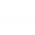## Recursion

#### Recursion is one of the most useful aspects of subroutines; that is the ability of the procedure or subroutine to call itself.

This article will use a rather ancient puzzle known as the Tower of Hanoi originated from Hindu.Diagram Tower of Hanoi
The rules of this puzzle are:

1. We are interested in moving the rings from A to B, perhaps using  C in the process.
2. Also by the rules of the game, rings are to be moved one at a time
3.  At no point may the larger ring be placed on top a smaller one.
This puzzle can be solved following these 7 steps:
• move A to  B;
• move A  to C;
• move B  to C;
• move A to  B;
• move C  to A;
• move C  to B;
• move A  to B;

### Algorithm

subroutine move N from A to B using C;
1. if N is 1 then print "move A to B";
2. else( If N is greater than 1) do the following:
2.1 call move N - 1 from  A to C using B;
2.2 call  move 1   from A to B using  C;
2.3 call move N - 1 from  C to B using A;
3. return

#### Algorithm

We have 3 rings on Peg A, therefore, our N = 3, which is greater than 1.
After the function; below are the 3 Steps our code follows:
P(3, A, C, B) means  call move 3 from A to B using C
Step1: P(N - 1, Beg, End, Aux)
Step2: P(1,       Beg,  Aux, End)
Step3: P(N - 1, Aux, Beg, End)

### Below also are the meaning of the variables selected:

P = denotes procedure/subroutine
N = numbers of ring
Beg = Initial stage of moving the ring
End = destination
Aux = auxiliary output through which we move rings

If N is greater than 1:
Step1  says: move top (N -1) rings from Beg to Aux peg.
Step2  says: move 1 ring from Beg to End peg.
Step3 says: move top (N-1) rings from Aux to End peg.

Let us see in code:
namespace TowerOfHanoi
{
class Program
{
static void Main(string[] args)
{
char beg_fromPeg = 'A';       // Begining of the tower in output where we move from
char dest_Peg = 'B';            //  end tower in output where we move to
char via_PegtoDest = 'C';   // auxilliary tower in output through which we move
int disks = 3;                    // number of disks to move around
solverHanoiTowers(disks, beg_fromPeg, via_PegtoDest, dest_toPeg);
}
private static void solverHanoiTowers(int n, char beg_fromPeg, char via_PegtoDest, char dest_toPeg)
{
if ( n == 1)
{

Console.WriteLine("move" + beg_fromPeg + "to" + dest_Peg);

}
else
{
solverHanoiTowers(n - 1, beg_fromPegdest_Pegvia_PegtoDest);
solverHanoiTowers(1, beg_fromPegvia_PegtoDest, dest_Peg);
solverHanoiTowers(n - 1, via_PegtoDest, beg_fromPegdest_Peg);

}

}
}
}

OUTPUT
move A to B;
move A to C;
move B to C;
move A to B;
move C to A;
move C to B;
move A to B;

These processes below is the sequential flow that moves all the rings in peg A to B recursively.

P( 3 , A, B, C)
/                                            1st Chain                    2nd Chain           3rd Chain
P(2, A, C, B) - Step 1,2,3.    P(2 , A, C, B)          P(1,A, B, C)= A to C    P(2, B, A, C)
/                                            /                                                                     /
P(1, A, B,  C)  - Step 2.        P(1, A, B, C) = A to C                               P(1,B,C,A)= B to A
/                                             /                                                                    /
P(2, B, A, C)  -  Step 1,2,3.   P(1, A, C, B)= A to B                              P(1,B,A,C)= B to C
/                                                                    /
P(1, C, A, B)= C to B                             P(1, A, B, C) = A to C

Here what happened is that we applied those 3 steps on P(3, A, B, C) and those are the chain we got out of it that gave us the accurate result in code.

Read: Class and Object -Object-Oriented Programming

1.Thanks for posting

1.You are welcome,I'm happy it helps you.

2.This is easy to understand tutorial, thanks for sharing.

1.3.Here's [a link](https://www.maxybyte.com/p/counter-in-vhdl-with-debouncer.html)! that might help